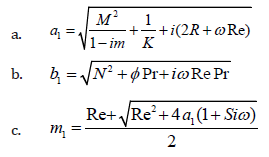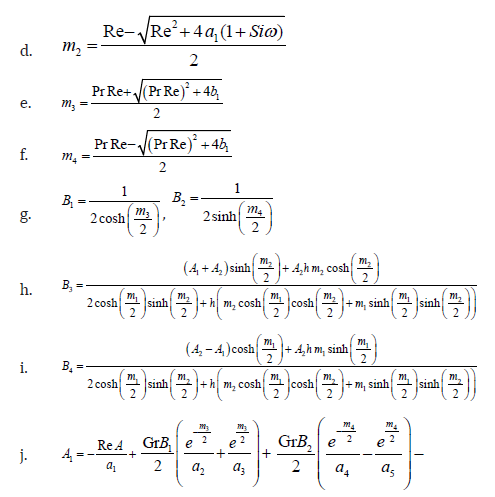## Friday 8 September 2023

### Lupine Publishers | Hall Effects on MHD Convective Flow Through a Porous Medium Between Two Vertical Plates in Slip Flow Regime

Lupine Publishers | Journal of Oceanography and Petrochemical Science

## Abstract

In this paper, a probe on the impact of Hall effects on the flow of an oscillatory convective MHD viscous, incompressible, radiating and electrically conducting second-grade fluid in a vertical porous rotating channel in a slip flow regime is carried out. The fluid is assumed to be gray, absorbing and emitting radiation in a non-scattering medium. It is presupposed that MHD flow is laminar and fully developed. In order to use the regular perturbation technique, the solutions of velocity and temperature distributions for the governing flow are obtained. The profiles of velocity and temperature are presented graphically. On the other hand, the skin friction coefficient and the Nusselt number are evaluated numerically and presented in the form of tables, examined and discussed for various values of the flow parameters that govern it.

Keywords: Hall effect; radiating fluid; MHD oscillatory flow; rotating channel; slip flow regime

## Introduction

Rapid efforts have been made to study the effects of various fields in porous media by both experimentally and theoretically. Flows through porous medium are of principal interest in the fields of agricultural engineering, underground water resources and seepage of water in riverbeds, filtration and purification processes in ocean engineering, petroleum technology to study the movement of natural gas, oil and water through the oil reservoirs. The way in which fluid flow through porous media is of great importance for the petroleum extraction processes. Most of the problems have considerable practical significance besides contributing more to the existing knowledge. Porous materials due to their inherent capability are widely used in Ocean engineering, Mechanical engineering, Medical appliances and Modern industrial fields. The practical applications in geophysics and engineering promote the study of flow in a rotating porous channel. Besides that, its applications are extended to food processing industry, the chemical processing industry, centrifugation filtration processes, and rotating machinery. In addition to that, the hydrodynamic rotating flow of electrically conducting viscous incompressible fluids has garnered adequate consideration due to its variegated applications in engineering and physics. In the domain of geophysics, it can be applied to measure and study position and velocities with respect to a fixed frame of reference on the surface of the earth, which rotates with respect to an inertial frame in the presence of its magnetic field. The area of geophysical dynamics, of late has become a significant branch of fluid dynamics due to the enormous interest towards environment and its concerns. As there is growing inquisitiveness to study astrophysics, the research on fluid dynamics has become vital as through it stellar and solar structures, interplanetary and interstellar matter, solar storms, etc. can be studied. In engineering, it finds its application in MHD generator ion propulsion, MHD bearing, MHD pumps, MHD boundary layer control of re-entry vehicles, etc. Several scholars [1-3] have studied such flows because of their varied importance and applications.
The process of heat transfer is used in the cooling of nuclear reactors, providing heat sink in turbine blades and aeronautics. There are countless, significant engineering and geophysical applications of channel flows through a porous medium: for example, in the field of agricultural engineering, for channel irrigation and for studying underground water resources; and in petroleum technology, to study the environment of natural gas, oil and water through the oil channels/reservoirs. The transient natural convection between two vertical walls with porous material having variable porosity has been studied by . In recent years, the effect of transversely applied magnetic field on the flow of an electrically conducting viscous fluid has been studied extensively owing to its astrophysics, geophysical, and engineering applications.  have studied MHD flow between the two parallel plates with heat transfer. When the strength of magnetic field is strong, one cannot overlook or negate the effect of Hall current. The rotating flow of an electrically conducting fluid in the presence of a magnetic field is encountered in geophysical and comical fluid dynamics. It is indispensable in solar physics involved in sunspot development. The Hall effect on unsteady MHD free and forced convection flow in a porous rotating channel has been investigated by several researchers, including [6- 9]. Radiative convective flows have gained the attention of many researchers in recent years. Radiation plays a crucial role in several engineering, environment and industrial processes: for example, heating and cooling chambers; fossil fuel combustion energy processes; astrophysical flows; and space vehicle re-entry.  studied radiation free convective flow through a porous medium  have analysed the radiation effect on MHD flow through a porous medium between infinite parallel plates in the presence of time-dependent suction.  have studied the radiation effect on the exact solution of free convective oscillatory flow through a porous medium in a rotating porous channel.

## Formulation and Solution of the Problem

Consider the flow of a viscous, incompressible, and electrically conducting second grade fluid through a porous medium bounded by two infinite vertical insulated plates at d distance apart, under the influence of a uniform transverse magnetic field normal to the channel and taking Hall current into account. We introduce a Cartesian co-ordinate system with the x-axis oriented vertically upward along the centreline of this channel, and the z-axis taken perpendicular to the planes of the plates, which is the axis of the rotation, and the entire system comprising of the channel and the fluid are rotating as a solid body about this axis with constant angular velocity Ω. A constant injection velocity w0 is applied at the plate z = −d / 2 and the same constant suction velocity, w0 , is applied at the plate z = d / 2 . The schematic diagram of the physical problem is shown in Figure 1. Since the plates of the channel occupying the planes z = ± d / 2 are of infinite extent, all the physical quantities depend upon z and t only. Under the Boussinesq approximation, the flow of the fluid through a porous medium in a rotating channel is governed by the following equation:When the strength of the magnetic field is very large, the generalized Ohm’s law is modified to include the Hall current, so that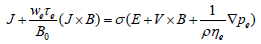(2.7)

In equation (2.5), the electron pressure gradient, the ion–slip effect, and the thermo-electric effect are neglected. We also assume that the electric field E=0 under assumption reduces to,where m =τe ωe is the Hall parameter.
On solving the equations (2.8) and (2.9),
we obtain,Using the equations (2.10) and (2.11), the equations (2.2) and (2.3) reduce: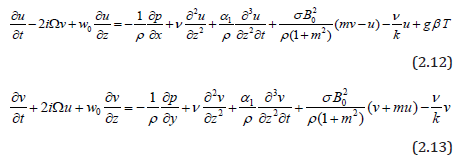Following Cogley et al. , the last term in the energy equation stands for radiative heat flux, which is given by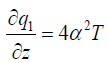(2.14)

whereα is the mean variation absorption coefficient.
Introducing non-dimensional variables,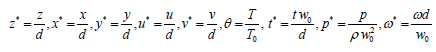Making use of the non-dimensional variables, the equations (2.12), (2.13) and (2.4) reduce to (with the asterisks being dropped)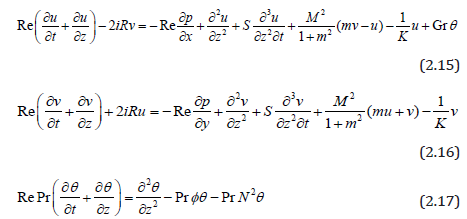The corresponding boundary conditions in non-dimensional form are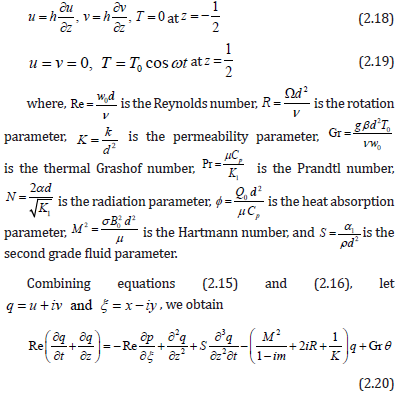We assume the flow under the influence of pressure gradient varying periodically with time,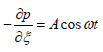(2.21)

Upon substitution of equation (2.21) into equation (2.20), we obtain(2.22)

The corresponding boundary conditions are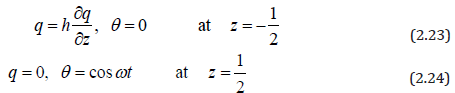In order to solve the equations (2.22) & (2.17) with the boundary conditions (2.23) & (2.24), following Choudary et al. , we assume a solution of the formSubstituting the equations (2.25) and (2.26) in (2.22) and (2.17) respectively yields the following equations: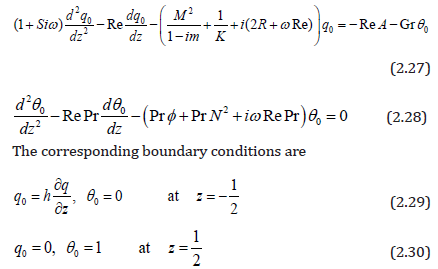Solving the equations (2.27) and (2.28) with respect to the boundary conditions (2.29) and (2.30), we obtain the following expressions for the velocity and the temperature.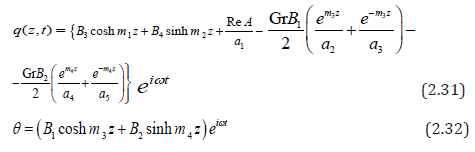The validity and the correctness of the present solution are verified by taking S = Gr = M = R= h = 0 and K →∞.
i.e., for the horizontal channel in the absence of rotation, slip flow and the condition of the ordinary medium so that2 . 3 3 )

This solution is reported by Schlichting and Gersten  for periodic variation of the pressure gradient along axis of the channel.
We find the skin friction τL at the left plate in terms of its amplitude and the phase angle as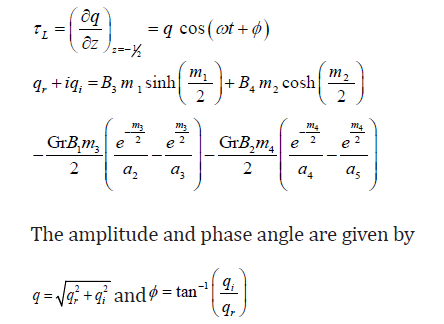The rate of heat transfer (NU), in terms of amplitude and the phase angle, can be obtained as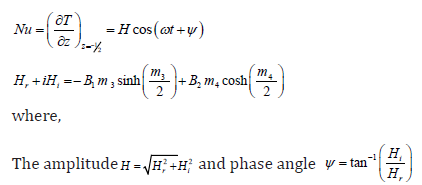All the contents used above have been mentioned in the Appendix.

## Results and Discussions

We have discussed the heat transfer on the flow of an oscillatory convective MHD viscous incompressible, radiating, and electrically conducting second grade fluid in a vertical porous rotating channel in slip flow regime, taking Hall current into account. The flow is governed by the non-dimensional parameters, namely, Re the Reynolds number, A the amplitude of pressure gradient, h the slip parameter, m the Hall parameter, R the rotation parameter, K the permeability parameter, Gr the thermal Grashof number, Pr the Prandtl number, N the Radiation parameter, φ1 the Heat absorption parameter, M the Hartmann number, S the second grade fluid parameter and ω the frequency of oscillation. The velocity profiles are depicted in Figures 2-5, while the temperature profiles are depicted in Figure 6. The skin friction and the Nusselt number are tabulated in Tables 1,2. For computational purposes, we are fixing the valuesRe = 2, t = 0.1 for the velocity profiles.

Figure 2:The velocity profiles for u and v against R, m and S with 1 Gr = 3,M = 0.5, Pr = 0.71, K = 0.5, A = 5, h = 0.2,φ1 = 0.1, N =1,ω =π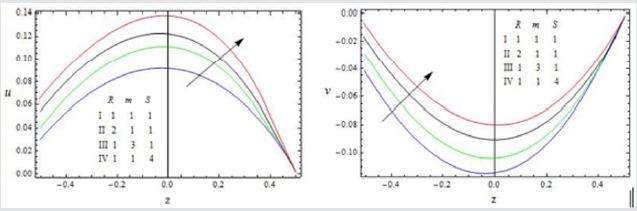Figure 3: The velocity profiles for u and v against Gr, K and A with 1 R =1,M = 0.5, Pr = 0.71, m =1, S =1, h = 0.2,φ1 = 0.1, N =1,ω =πFigure 4: The velocity profiles for u and v against φ1 , h and ω with Gr = 3,M = 0.5, Pr = 0.71, K = 0.5, S =1,m =1, R =1, N =1, A = 5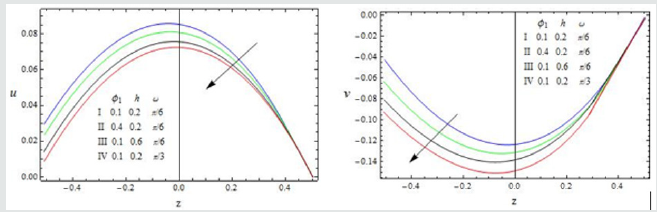Figure 5: The velocity profiles for u and v against M, Pr and N with 1 Gr = 3, S =1,m =1, R =1, K = 0.5, A = 5,h = 0.2,φ = 0.1,ω =π / 6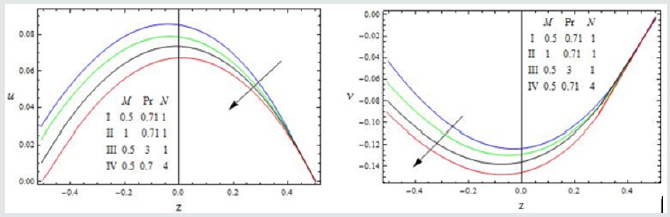The effect of the rotation number R, the Hall parameter m, and the second-grade fluid parameter S on the velocity profile is shown in Figure 2. We notice that the magnitudes of velocity components u and v enhance with increasing values of R, m and S throughout the fluid region. A similar behaviour is observed for increasing thermal Grashof number Gr, permeability parameter K and the amplitude of the pressure gradient A Figure 3. It is observed from these Figures 4,5 that the velocity profile is diminished with the increase of all these parameters: the heat absorption parameter φ1 , the slip parameter h, the frequency of oscillationω , the Hartmann number M, the Prandtl number Pr, and the radiation parameter N. That is, it starts decreasing near the left plate of the channel. The variations in the temperature profile are presented in Figure 6. It is observed that the temperature profiles decrease with the increasing Reynolds number Re and the Prandtl number Pr. Likewise, the temperature profile is diminished with increasing the radiation parameter N and the frequency of oscillation ω .The skin friction and Nusselt number are evaluated analytically and tabulated in Tables 1,2. The amplitude and phase angle of the skin friction are shown in Table 1. The negative values in this table indicate that there is always a phase lag, and this phase goes on increasing with increasing frequency of oscillation. We notice that the amplitude |q| and phase angle γ of frictional force are enhanced with increasing the parameters S and A, and diminished with increasing h, Pr and R. The amplitude of the frictional force increases, and the phase angle of frictional force reduces with increasing the parameters K, Gr, m and φ1. The opposite behaviour is observed with increasing M, N and ω . Likewise, the amplitude of the Nusselt number and the magnitude of the phase angle increases with Re, Pr, N and φ1. The amplitude of the Nusselt number increases and the phase angle decreases with increasing ω .

## Conclusions

The flow and heat transfer of an oscillatory convective MHD viscous incompressible, radiating and electrically conducting second grade fluid in a vertical porous rotating channel in slip flow regime and taking Hall current into account has been discussed.

1. The resultant velocity enhances with increasing R, m, Gr, K, A and S throughout the fluid region.

2. The velocity profile is diminished with the increase of φ1 , h, ω , M, Pr and N.

3. The temperature profiles decrease with the increasing Re, N, Prand ω .

4. The amplitude and phase angle of frictional force are enhanced with increasing S and A, and diminished with increasing h, Pr and R.

5. The amplitude of the frictional force increases, and the phase angle of frictional force reduces with increasing the parameters K, Gr, m and φ1

. The opposite behaviour is observed with increasing M, N and ω .

6. The amplitude of the Nusselt number and the magnitude of the phase angle increase with Re, Pr, N and φ1 . The amplitude of the Nusselt number increases and the phase angle decreases with increasing ω .

7. Nomenclature:

1(u, v): The velocity components along x and y directions

2P: The modified pressure

3t: Time

4: Specific heat

5B0: Electromagnetic induction

6g: Acceleration due to gravity

7K1: Thermal conductivity

8K: Permeability of the porous medium

9. Q0 : Heat absorption

10. T: Temperature of the fluid

11. f1 : Maxwell’s reflection co-efficient

12. μ : Co-efficient of viscosity

13. L : Mean free path

14. T0 : Mean temperature

15. w0 : Suction velocity

17. q: Complex velocity

18. M: Hartmann number

19. K: Permeability parameter

20. S: Second grade fluid parameter

21. E: Ekman number

22. Gr: Thermal Grashof number

23. Pr: Prandtl number

24. m: Hall parameter

7.1. Greek symbols

25. α : The mean variation absorption coefficient 26. α1: Normal stress moduli 27. θ : Dimension less temperature 28. Ù : Angular velocity 29. σ : Electrical conductivity of the fluid 30. ρ : Density of the fluid 31. ν : Kinematic viscosity 32. ω : The frequency of oscillation 33. β : The coefficient of volume expansion 34. ωe: The electron frequency 35. τe : The electron charge

7.2. Sub scripts

36. E: Electron

## Appendix